# Linear Search Program in Python

This article is created to cover some programs in Python that performs linear search. Linear search is very basic and simplest technique to search an element. Here are the list of programs on linear search:

• Linear search based on 10 elements or numbers entered by user
• Linear search based on n elements entered by user
• Linear search along with duplicate elements

Before proceeding the program given below, if you want to get some idea about the algorithm used, then refer to Linear Search Algorithm & Example article to get every required things. You can also proceed directly. It will not confuse you. It's very simple.

## Linear Search based on 10 Elements

The question is, write a Python program to perform linear search on 10 elements. The program given below is answer to this question:

```print("Enter 10 Numbers: ")
arr = []
for i in range(10):
arr.insert(i, int(input()))
print("Enter the Number to Search: ")
num = int(input())
for i in range(10):
if num==arr[i]:
index = i
break
print("\nNumber Found at Index Number: ")
print(index)```

Here is its sample run: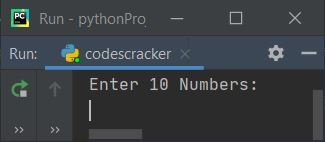Now supply the input say 10, 20, 30, 40, 50, 60, 70, 80, 90, 100 as ten numbers, then 70 as number to search and print its index number: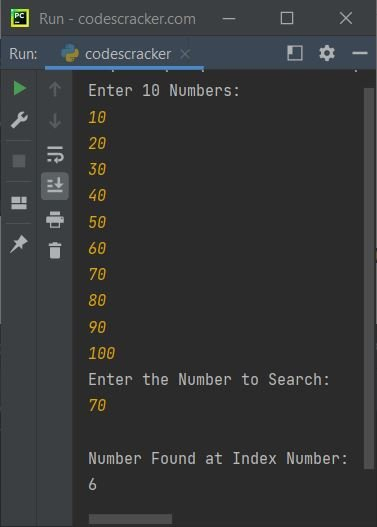## Linear Search based on n Elements

This is the modified version of previous program. This program allows user to define the size of list along with its elements. Let's have a look at the program and its sample output to get clear understanding about this section:

```print(end="Enter the Size: ")
arrSize = int(input())
print("Enter " +str(arrSize)+ " Elements: ")
arr = []
for i in range(arrSize):
arr.append(input())
print("Enter an Element to Search: ")
elem = input()
chk = 0
for i in range(arrSize):
if elem==arr[i]:
index = i
chk = 1
break
if chk==1:
print("\nElement Found at Index Number: " + str(index))
else:
print("\nElement doesn't found!")```

Here is its sample run with user input, 5 as size, c, o, d, e, s as five elements, e as element to search: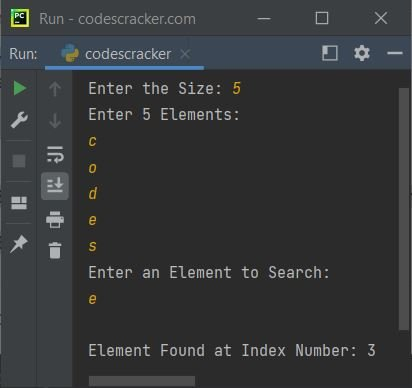Here is another sample run with user input, 5 as size, 1, 2, 3, 4, 5 as five elements, 10 as element to search: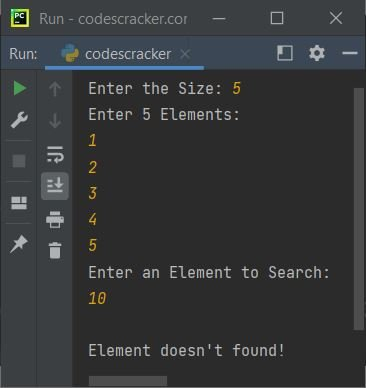## Linear Search with Duplicate Elements

This is the last program of this article's linear search program's list. This program find and prints duplicate elements too. For example, if there are six elements say 1, 2, 3, 2, 4, 2. And user wants to search the element 2 from the list. Since 2 presents three times. Therefore this program prints all the three positions of the element.

```print(end="Enter the Size: ")
arrSize = int(input())
print("Enter " +str(arrSize)+ " Elements: ")
arr = []
for i in range(arrSize):
arr.append(input())
print("Enter an Element to Search: ")
elem = input()
k = 0
index = []
for i in range(arrSize):
if elem==arr[i]:
index.insert(k, i)
k = k+1
if k==0:
print("\nElement doesn't found!")
else:
if k==1:
print("\nElement Found at Index Number: " + str(index))
else:
print(end="\nElement Found at Index: ")
indexLen = len(index)
for i in range(indexLen):
print(end=str(index[i])+" ")
print()```

Here is its sample run with user input, 6 as size, 1, 2, 3, 2, 4, 2 as six elements, then 2 as element to find and print its index numbers of all occurrences: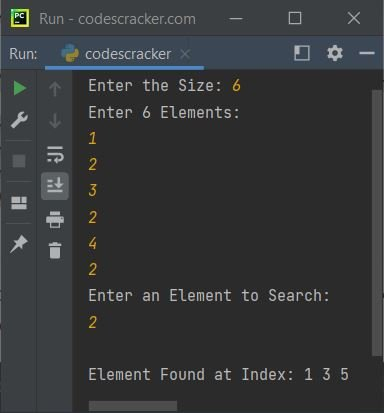Python Online Test

« Previous Program Next Program »